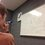In Need of a Proof

Main post link -> http://en.wikipedia.org/wiki/Multiset#Recurrence_relation

I recently came across this interesting equation: $\displaystyle \sum_{k=0}^{n} \binom{m+k-1}{k} = \binom{n+m}{n}$ Wikipedia said that the result was related to the multiset, to which I have posted a link to in the title of the discussion. I still can't understand, though, why this equation is true. Can anyone figure it out?Note by Bob Krueger
6 years, 3 months ago

This discussion board is a place to discuss our Daily Challenges and the math and science related to those challenges. Explanations are more than just a solution — they should explain the steps and thinking strategies that you used to obtain the solution. Comments should further the discussion of math and science.

When posting on Brilliant:

• Use the emojis to react to an explanation, whether you're congratulating a job well done , or just really confused .
• Ask specific questions about the challenge or the steps in somebody's explanation. Well-posed questions can add a lot to the discussion, but posting "I don't understand!" doesn't help anyone.
• Try to contribute something new to the discussion, whether it is an extension, generalization or other idea related to the challenge.
• Stay on topic — we're all here to learn more about math and science, not to hear about your favorite get-rich-quick scheme or current world events.

MarkdownAppears as
*italics* or _italics_ italics
**bold** or __bold__ bold

- bulleted
- list

• bulleted
• list

1. numbered
2. list

1. numbered
2. list
Note: you must add a full line of space before and after lists for them to show up correctly
paragraph 1

paragraph 2

paragraph 1

paragraph 2

> This is a quote
This is a quote
# I indented these lines
# 4 spaces, and now they show
# up as a code block.

print "hello world"
# I indented these lines
# 4 spaces, and now they show
# up as a code block.

print "hello world"
MathAppears as
Remember to wrap math in $$...$$ or $...$ to ensure proper formatting.
2 \times 3 $2 \times 3$
2^{34} $2^{34}$
a_{i-1} $a_{i-1}$
\frac{2}{3} $\frac{2}{3}$
\sqrt{2} $\sqrt{2}$
\sum_{i=1}^3 $\sum_{i=1}^3$
\sin \theta $\sin \theta$
\boxed{123} $\boxed{123}$

Sort by:

There's an easy direct proof, if you consider where the binomial coefficients are in Pascal's triangle.

As an additional hint, ${m-1 \choose 0} = 1 = {m \choose 0}$.

Staff - 6 years, 3 months ago

The hockey stick identity! Thanks!

- 6 years, 3 months ago

I guess the easiest way to prove this is to use induction, i.e., you just need to show ${m+n}\choose {n}+ {m+n}\choose {n+1}={m+n+1}\choose {n+1}$. It's not hard to show this equality using the definition of binomial expansion. As always a induction proof is not as satisfying as a conceptual explanation.

- 6 years, 3 months ago

Well if you need a conceptual proof here it is:
The Left hand side of the equation(LHS) can be recognized as the Coefficient of $x^m$ in $\sum_{k=0}^n (1+x)^{(m+k-1)}*x^{m-k}$ (Such equations can be constructed with little analysis)....

This can be treated as a geometric progression(GP) and the well know formula for a GP can be used to get the required result as coefficient of $x^m$ in $(1+x)^{(m+n)}*x^{(m-n)}$ ....
which is ${(m+n) \choose n}$ ....
I have neglected the simplification of the GP sum you can Wikipedia for the sum of the GP formula and simplify it yourself :)

- 6 years, 3 months ago

Really late but here goes: (Induction on n)

We establish a base case as $n=0$:

$\sum_{i=0}^{0} \dbinom{m+i-1}{i} = \dbinom{m}{0}$

$\Rightarrow$ $\dbinom{m-1}{0} = \dbinom{m}{0} \Rightarrow 1=1$

The base case clearly holds. Now the inductive case for $n=k$:

$\sum_{i=0}^{k} \dbinom{m+i-1}{i} = \dbinom{m+k}{k}$

$\Rightarrow$ $\dbinom{m+k}{k+1} + \sum_{i=0}^{k} \dbinom{m+i-1}{i} =\dbinom{m+k}{k+1} +\dbinom{m+k}{k}$ (By hypothesis)

$\Rightarrow$ $\sum_{i=0}^{k+1} \dbinom{m+i-1}{i} = \frac{(m+k)!}{(k+1)!(m-1)!} + \frac{(m+k)!}{k!m!}$

$\Rightarrow$ $\sum_{i=0}^{k+1} \dbinom{m+i-1}{i} = \frac{(m+k)!(m+k+1)}{(k+1)!m!}$

$\Rightarrow$ $\sum_{i=0}^{k+1} \dbinom{m+i-1}{i} = \dbinom{m+k+1}{k+1}$

So we may conclude that the statement holds for the base case and that if the statement holds for some $n=k$, then it holds for $n=k+1$. The proof follows by induction.

QED

- 5 years, 2 months ago

This can be easily verified by forming a recursion i hope...

- 6 years, 3 months ago